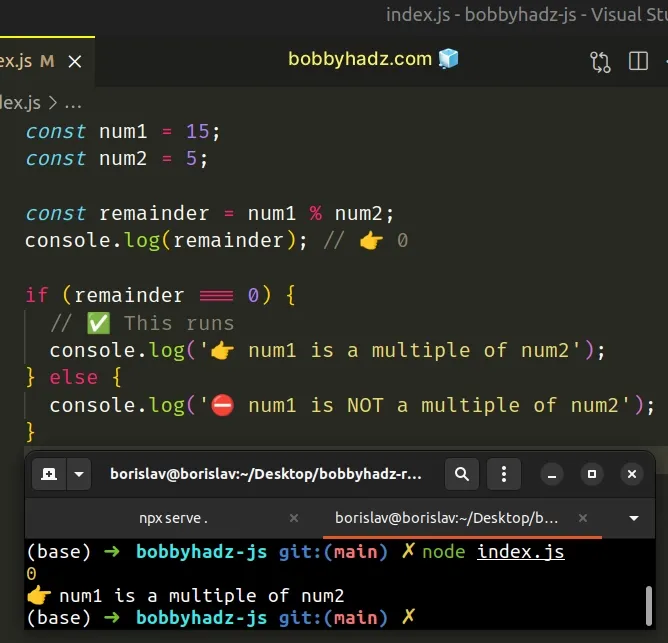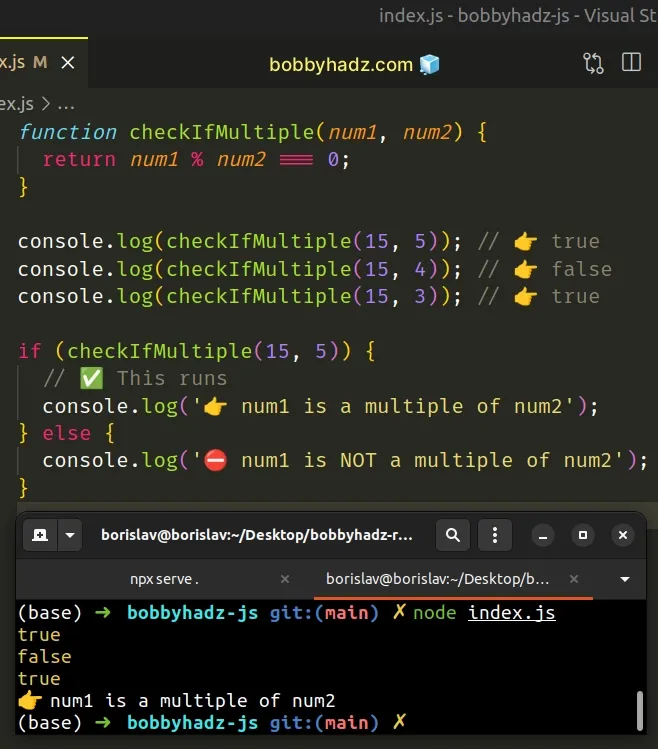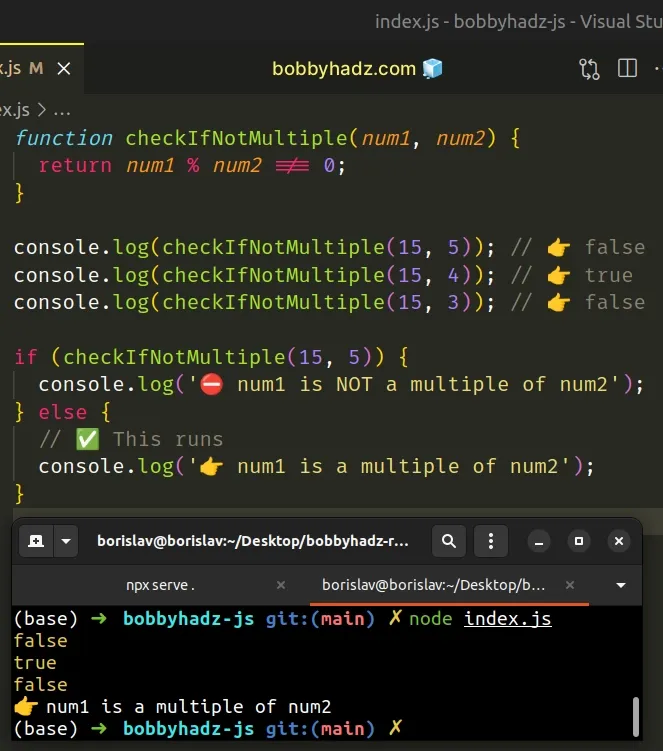# Check if one Number is Multiple of another in JavaScriptLast updated: Dec 26, 2022
2 min## #Check if One Number is Multiple of Another

Use the modulo `%` operator to check if one number is a multiple of another number.

The operator returns the remainder when one number is divided by another number. The remainder will only be zero if the first number is a multiple of the second.

index.js
```Copied!```const num1 = 15;
const num2 = 5;

const remainder = num1 % num2;
console.log(remainder); // 👉️ 0

if (remainder === 0) {
// ✅ This runs
console.log('👉️ num1 is a multiple of num2');
} else {
console.log('⛔️ num1 is NOT a multiple of num2');
}
``````We used the modulo operator to get the remainder of dividing one number by another.

If the remainder after dividing the first by the second number is `0`, then the first number is a multiple of the second.

Here are some more examples of using the modulo `%` operator.

index.js
```Copied!```console.log(13 % 5); // 👉️ 3
console.log(12 % 5); // 👉️ 2
console.log(11 % 5); // 👉️ 1
console.log(10 % 5); // 👉️ 0
``````

The number `10` is a multiple of `5`, so the division has a remainder of `0`.

The modulo `%` operator always takes the sign of the first number (the dividend).

index.js
```Copied!```console.log(-13 % 5); // 👉️ -3
console.log(12 % -5); // 👉️ 2
``````

You might be wondering what happens if we get a remainder of `-0`.

Would the `if` condition fail because we only check for `0`.

index.js
```Copied!```console.log(-15 % 3); // 👉️ -0
``````

However, `0` is equal to `-0` in JavaScript so our condition would still work.

index.js
```Copied!```console.log(0 === -0); // 👉️ true
``````

## #Creating a reusable function

If you need to check if a number is a multiple of another number often, extract the logic into a reusable function.

index.js
```Copied!```function checkIfMultiple(num1, num2) {
return num1 % num2 === 0;
}

console.log(checkIfMultiple(15, 5)); // 👉️ true
console.log(checkIfMultiple(15, 4)); // 👉️ false
console.log(checkIfMultiple(15, 3)); // 👉️ true

if (checkIfMultiple(15, 5)) {
// ✅ This runs
console.log('👉️ num1 is a multiple of num2');
} else {
console.log('⛔️ num1 is NOT a multiple of num2');
}
``````The `checkIfMultiple()` function takes 2 numbers as parameters and checks if the first number is a multiple of the second.

## #Checking if a number is not a multiple of another number

If you need to check if a number is not a multiple of another number, check if there is a remainder after using the modulo operator.

index.js
```Copied!```function checkIfNotMultiple(num1, num2) {
return num1 % num2 !== 0;
}

console.log(checkIfNotMultiple(15, 5)); // 👉️ false
console.log(checkIfNotMultiple(15, 4)); // 👉️ true
console.log(checkIfNotMultiple(15, 3)); // 👉️ false

if (checkIfNotMultiple(15, 5)) {
console.log('⛔️ num1 is NOT a multiple of num2');
} else {
// ✅ This runs
console.log('👉️ num1 is a multiple of num2');
}
``````The `checkIfNotMultiple()` function takes 2 numbers as parameters and checks if the first number is not a multiple of the second.

I wrote a book in which I share everything I know about how to become a better, more efficient programmer.You can use the search field on my Home Page to filter through all of my articles.